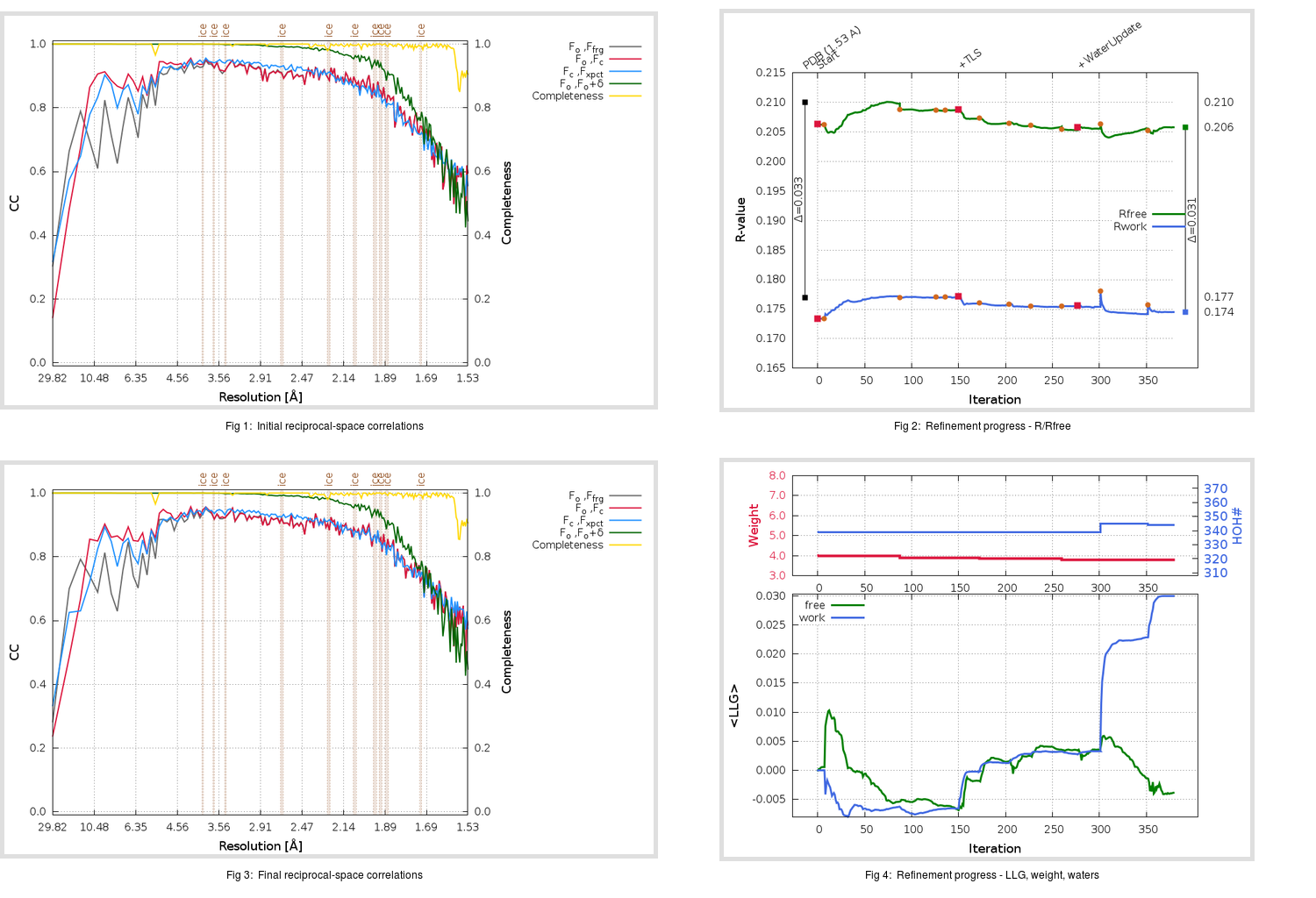Content:

## Deposited

` `
 Date deposited Date data collection Resolution R, Rfree 20200315 20200305 1.53 0.1750 0.2100

Molprobity (CCP4 7.0 version) summary:

```Ramachandran outliers =   0.33 %
favored =  97.35 %
Rotamer outliers      =   0.38 %
C-beta deviations     =     0
Clashscore            =   6.11
RMS(bonds)            =   0.0134
RMS(angles)           =   1.86
MolProbity score      =   1.46
Resolution            =   1.53
R-work                =   0.1750
R-free                =   0.2100
```

```Number of waters      =   339

<B> (all atoms) =   21.20 ( sd =    9.56 ) for       2743 non-hydrogen atoms
<B>   (protein) =   19.55 ( sd =    8.17 ) for       2370 non-hydrogen atoms
<B>     (water) =   32.02 ( sd =   11.32 ) for        339 non-hydrogen atoms
<B>    (others) =   27.98 ( sd =    7.84 ) for         34 non-hydrogen atoms

B min/max       (all non-hydrogen atoms) =    9.99 /  131.81
B min/max   (protein non-hydrogen atoms) =    9.99 /   68.35
B min/max     (water non-hydrogen atoms) =   11.52 /  131.81
B min/max     (other non-hydrogen atoms) =   17.51 /   45.33
```

## BUSTER (re-)refinement

` `

Molprobity (CCP4 7.0 version) summary:

```Ramachandran outliers =   0.33 %
favored =  98.34 %
Rotamer outliers      =   1.52 %
C-beta deviations     =     0
Clashscore            =   2.11
RMS(bonds)            =   0.0114
RMS(angles)           =   1.56
MolProbity score      =   1.12
Resolution            =   1.53
R-work                =   0.1745
R-free                =   0.2058
```

```Number of waters      =   344

<B> (all atoms) =   22.43 ( sd =    8.77 ) for       2748 non-hydrogen atoms
<B>   (protein) =   20.60 ( sd =    7.02 ) for       2370 non-hydrogen atoms
<B>     (water) =   34.36 ( sd =   10.11 ) for        344 non-hydrogen atoms
<B>    (others) =   28.60 ( sd =    7.89 ) for         34 non-hydrogen atoms

B min/max       (all non-hydrogen atoms) =   11.24 /   71.98
B min/max   (protein non-hydrogen atoms) =   11.62 /   63.96
B min/max     (water non-hydrogen atoms) =   11.24 /   71.98
B min/max     (other non-hydrogen atoms) =   20.62 /   36.25
```

Refinement progression:Results:

` `
 File Remark 5RFU_aB_refine.01_03_refine.pdb.gz exact refinement commands are in header 5RFU_aB_refine.01_03_refine.mtz.gz including original deposited data and several re-refinement map coefficients 5RFU_aB_refine.01_03_BUSTER_model.cif.gz including any non-standard compound restraints 5RFU_aB_refine.01_03_BUSTER_refln.cif.gz